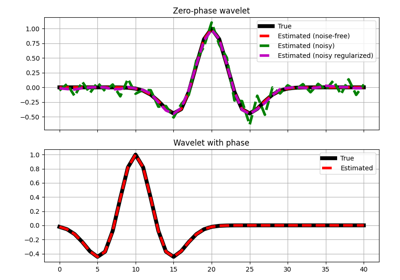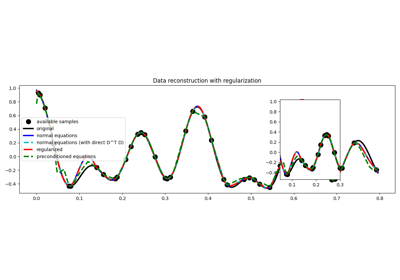# pylops.optimization.leastsquares.PreconditionedInversion¶

pylops.optimization.leastsquares.PreconditionedInversion(Op, P, data, x0=None, returninfo=False, **kwargs_solver)[source]

Preconditioned inversion.

Solve a system of preconditioned equations given the operator Op and a preconditioner P.

Parameters: Op : pylops.LinearOperator Operator to invert P : pylops.LinearOperator Preconditioner data : numpy.ndarray Data x0 : numpy.ndarray Initial guess returninfo : bool Return info of LSQR solver **kwargs_solver Arbitrary keyword arguments for chosen solver (scipy.sparse.linalg.lsqr and pylops.optimization.solver.cgls are used as default for numpy and cupy data, respectively) Returns ——- xinv : numpy.ndarray Inverted model. istop : int Gives the reason for termination 1 means $$\mathbf{x}$$ is an approximate solution to $$\mathbf{d} = \mathbf{Op}\mathbf{x}$$ 2 means $$\mathbf{x}$$ approximately solves the least-squares problem itn : int Iteration number upon termination r1norm : float $$||\mathbf{r}||_2^2$$, where $$\mathbf{r} = \mathbf{d} - \mathbf{Op}\mathbf{x}$$ r2norm : float $$\sqrt{\mathbf{r}^T\mathbf{r} + \epsilon^2 \mathbf{x}^T\mathbf{x}}$$. Equal to r1norm if $$\epsilon=0$$

RegularizedInversion
Regularized inversion
NormalEquationsInversion
Normal equations inversion

Notes

Solve the following system of preconditioned equations given the operator $$\mathbf{Op}$$, a preconditioner $$\mathbf{P}$$, the data $$\mathbf{d}$$

$\mathbf{d} = \mathbf{Op} (\mathbf{P} \mathbf{p})$

where $$\mathbf{p}$$ is the solution in the preconditioned space and $$\mathbf{x} = \mathbf{P}\mathbf{p}$$ is the solution in the original space.

## Examples using pylops.optimization.leastsquares.PreconditionedInversion¶Wavelet estimation03. Solvers